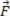Mechanics

# Work is the amount of energy expended in performing an activity, and its intensity is determined by the product of force and displacement.

Let’s see the figure below. In it, we will consider that a constant forceof magnitude acts on the block, which we will consider as a material point, causing it to undergo a displacement d .

Still based on the figure above, we can determine the work done by the force (τ) during the displacement, taking the product of the force applied at the material point by the displacement suffered by the material point and the cosine of the angle that forms between the orientations of the vector force and displacement. The equation that allows us to calculate the work done is the following:

τ = Fd .cosθ

A study of the equation that defines the work allows us to write the following:

If 0° ≤ θ <90° , the work done by the force is positive, and it is done by the force whose component F x acts in the direction of displacement, that is, the force is favoring the displacement in which it is acting.

If 90°< θ ≤180° , the work done is negative, and it is done by the force whose component F x acts in the opposite direction to the displacement, that is, the force is hindering the displacement of the material point on which it is acting.

SI units of work – the joule ( J )

If the force of magnitude F = 1 N is exerted in the same direction as the displacement of magnitude d = 1m, the angle being θ = 0°, cos 0° = 1, then the work done by this force is:

τ= 1N.1m .1
τ = 1Nm

Remembering that the product Nm is called joule ( J ) in honor of James Prescott Joule .